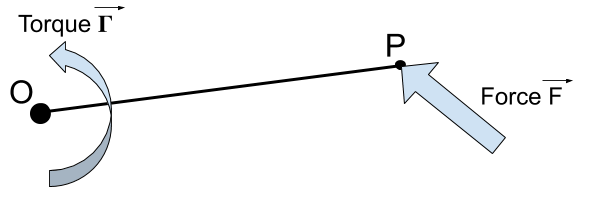Question #193

# What is the general formula to calculate the torque generated by a given force?

Merged questions
AnswerThe general formula to calculate the torque generated by a given force is the cross product of the force ﻿﻿ and vector ﻿﻿ :

﻿﻿

With :

• ﻿﻿ is the torque vector expressed in newtons-meters [N.m]
• ﻿﻿ is the force vector expressed in newtons [N]
• ﻿﻿ is the vector from rotation center ﻿﻿ to the point ﻿﻿ where the force ﻿﻿ is applied

3 events in history
Answer by Alphonsio 09/18/2020 at 05:48:39 PMThe general formula to calculate the torque generated by a given force is the cross product of the force ﻿﻿ and vector ﻿﻿ :

﻿﻿

With :

• ﻿﻿ is the torque vector expressed in newtons-meters [N.m]
• ﻿﻿ is the force vector expressed in newtons [N]
• ﻿﻿ is the vector from rotation center ﻿﻿ to the point ﻿﻿ where the force ﻿﻿ is applied

Answer by Alphonsio 09/18/2020 at 05:42:05 PMThe general formula to calculate the torque generated by a given force is the cross product of the force ﻿﻿ and vector ﻿﻿ :

﻿﻿

With :

• ﻿﻿ is the torque vector expressed in newtons-meters [N.m]
• ﻿﻿ is the force vector expressed in newtons [N]
• ﻿﻿ is the vector from rotation center ﻿﻿ to the point ﻿﻿ where the force ﻿﻿ is applied

Question by Alphonsio 09/18/2020 at 05:38:40 PM
What is the general formula to calculate the torque generated by a given force?

Icons proudly provided by Friconix.